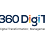## Thursday, October 24, 2019

Published October 24, 2019 by with 4 comments

# Compound Interest is Amazing

If it takes 40 years for a $100,000 investment to grow to$1,000,000, how long does it take it to grow an additional $1,000,000? It feels non-intuitive, but the answer is approximately 12 years. Why is that the case? The summary is that things like investments grow exponentially, so the time to make a percentage gain is constant. In this case, going from$100,000 to $1,000,000 means it's now 10 times as large while going from$1,000,000 to $2,000,000 means it's now 2 times as large, so it takes much less time. Phrased slightly differently, for a fixed interest rate, growing from$500 to $1,000 takes the same amount of time as growing from$5,000,000 to $10,000,000. #### Cool plots The first plot assumes an initial investment on the x-axis, and shows the years required for it to grow by$100,000 on the y-axis:

It is just so much faster with $1,000,000 than with$100,000.

The second plot shows the value of an initial $100,000 investment over time, and shades the periods required for$100,000 gains to show how much smaller it is later in the growth:

How does this all work?

#### Math behind this

The first problem said it took 40 years to go from $100,000 to$1,000,000 and wants to know how long it will take to go from $1,000,000 to$2,000,000. That is, if it takes 40 years to increase by a factor of 10, how long does it take to increase by a factor of 2? The equation we'll need is:
$$(1 + rate)^{time} = gain$$
First, we need the interest rate that yields a 10x increase in 40 years:
$$(1 + rate)^{40} = 10$$ $$40*log(1 + rate) = log(10)$$ $$log(1 + rate) = \frac{log(10)}{40}$$ $$rate = 10^{\frac{log(10)}{40}} - 1$$ $$rate \approx 0.059$$
So the interest rate is roughly 5.9%. Now we just see how long it takes to double with that interest rate:
$$(1.059)^{t} = 2$$ $$t*log(1.059) = log(2)$$ $$t \approx 12$$
Since our time is in years here, that means that it takes approximately 12 years to double if it takes 40 years to increase by 10x. From basically the same math as above, there's a simple relationship between increases:
$$\frac{t2}{t1} = \frac{log(gain 2)}{log(gain 1}$$
Testing it out, for gains of 10 and 2, we get that t2/t1 is ~3.3, and 40 / 12 ~ 3.3. Thus, a much faster way to solve that original problem is just:
$$\frac{40}{t} = \frac{log(10)}{log(2)}$$ $$t = 40*\frac{log(2)}{log(10)}$$ $$t \approx 12$$
And that's it...compound interest makes money grow really quickly (debt also). A bunch of idioms reference this, but it's cool that it's so easy to demonstrate.

1.I like viewing web sites which comprehend the price of delivering the excellent useful resource free of charge. I truly adored reading your posting. Thank you!
data science course

2.Thank you for taking the time and sharing this information with us. It was indeed very helpful and insightful while being straight forward and to the point…

3.🔥 IPRO356 Easy to play, pay for real 💰 keep withdrawing
🎰 Slot game, low capital, heavy break
🎲 Live casino with beautiful girls
⚽️ Bet on all types of sports with higher odds than anyone else

✨ Randomly give Jackpot prizes to players every hour.
💸 5000-10,000 baht per prize over 100 awards
🔰 Nowhere dares to get as much as this again

4.I wanted to leave a little comment to support you and wish you a good continuation. Wishing you the best of luck for all your blogging efforts.